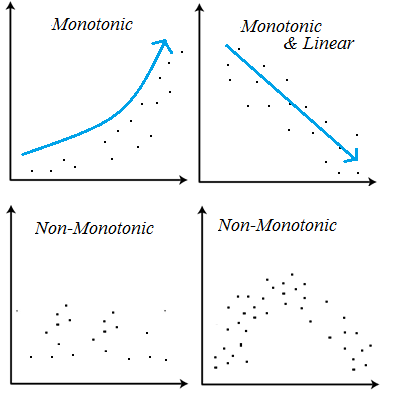# Monotonic Relationship: Definition

Share on

Statistics Definitions > Monotonic Relationship

## What is a Monotonic Relationship?

Monotonic relationships are where:

• One variable increases and the other increases. Or,
• One variable decreases and the other decreases.This increase (or decrease) doesn’t have to happen at the same rate. For example, the data in the image b above is monotonic and linear. Linear relationships are monotonic, but not all monotonic relationships are linear (as shown in image a).

• Monotonic variables increase (or decrease) in the same direction, but not always at the same rate.
• Linear variables increase (or decrease) in the same direction at the same rate.

If an increase in the independent variable causes a decrease in the dependent variable (image b), this is called a monotonic inverse relationship. An inverse relationship is the same thing as a negative correlation. A monotonic direct relationship is where an increase in the independent variable causes an increase in the dependent variable (image a). In other words, there’s a positive correlation between the data.

## Tests

Before running a test, you should make a scatter plot first to view the overall pattern of your data.

The strength and direction of a monotonic relationship between two variables can be measured by the Spearman Rank-Order Correlation. If your variables are monotonic and linear, a more appropriate test might be Pearson’s Correlation as long as the assumptions for Pearson’s are met. For example, if your data is highly skewed, has high kurtosis, or is heteroscedastic, you cannot use Pearson’s. You can, however, still use Spearman’s, which is a non-parametric test.

Values for Spearman’s range from 0-1 to 1, where:

• +1 = a perfect monotonically increasing relationship.
• -1 = a perfect monotonically decreasing relationship.
• 0 = not monotonic.

As correlation is an measure of association, you can also think of the results in terms of effect size:

• .00-.19: very weak.
• .20-.39: weak.
• .40-.59: moderate.
• .60-.79: strong.
• .80-1.0: very strong.
CITE THIS AS:
Stephanie Glen. "Monotonic Relationship: Definition" From StatisticsHowTo.com: Elementary Statistics for the rest of us! https://www.statisticshowto.com/monotonic-relationship/
------------------------------------------------------------------------------

Need help with a homework or test question? With Chegg Study, you can get step-by-step solutions to your questions from an expert in the field. Your first 30 minutes with a Chegg tutor is free!